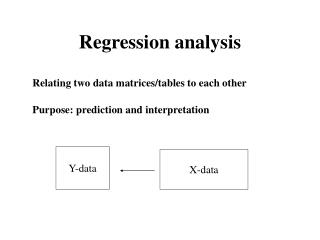Download PresentationRegression analysisRegression analysis - PowerPoint PPT Presentation

Download PresentationRegression analysis
An Image/Link below is provided (as is) to download presentation

Download Policy: Content on the Website is provided to you AS IS for your information and personal use and may not be sold / licensed / shared on other websites without getting consent from its author. While downloading, if for some reason you are not able to download a presentation, the publisher may have deleted the file from their server.

- - - - - - - - - - - - - - - - - - - - - - - - - - - E N D - - - - - - - - - - - - - - - - - - - - - - - - - - -
Presentation Transcript

1. Regression analysis Relating two data matrices/tables to each other Purpose: prediction and interpretation Y-data X-data

2. Typical examples • Spectroscopy: Predict chemistry from spectral measurements • Product development: Relating sensory to chemistry data • Marketing: Relating sensory data to consumer preferences

3. Topics covered • Simple linear regression • The selectivity problem: a reason why multivariate methods are needed • The collinearity problem: a reason why data compression is needed • The outlier problem: why and how to detect

4. Simple linear regression • One y and one x. Use x to predict y. • Use a linear model/equation and fit it by least squares

5. Data structure X-variable Y-variable 2 4 1 . . . 7 6 8 . . . Objects, same number in x and y-column

6. Least squares (LS) used for estimation of regression coefficients y y=b0+b1x+e b1 b0 x Simple linear regression

7. Model Regression analysis Data (X,Y) Future X Prediction Regression analysis Interpretation Outliers? Pre-processing

8. The selectivity problem A reason why multivariate methods are needed

9. Can be used for several Y’s also

10. Multiple linear regression • Provides • predicted values • regression coefficients • diagnostics • If there are many highly collinear variables • unstable regression equations • difficult to interpret coefficients: many and unstable

11. Collinearity, the problem of correlated X-variable y=b0+b1x1+b2x2+e Regression in this case is fitting a plane to the data (open circles) The two x’s have high correlation Leads to unstable equation/plane (in the direction with little variability)

12. Possible solutions • Select the most important wavelengths/variables (stepwise methods) • Compress the variables to the most dominating dimensions (PCR, PLS) • We will concentrate on the latter (can be combined)

13. Data compression • We will first discuss the situation with one y-variable • Focus on ideas and principles • Provides regression equation (as above) and plots for interpretation

14. Model for data compression methods X=TPT+E Centred X and y y=Tq+f T-scores, carrier of information from X to y P,q –loadings E,f – residuals (noise)

15. x3 PCA to compress data x2 ti x1 y q t-score Regression by data compression PC1 Regression on scores

16. x1 x2 MLR y x3 x4 x1 t1 x2 PCR y t2 x3 x4 x1 t1 y x2 PLS x3 t2 x4

17. PCR and PLS For each factor/component • PCR • Maximize variance of linear combinations of X • PLS • Maximize covariance between linear combinations of X and y Each factor is subtracted before the next is computed

18. Principal component regression (PCR) • Uses principal components • Solves the collinearity problem, stable solutions • Provides plots for interpretation (scores and loadings) • Well understood • Outlier diagnostics • Easy to modify • But uses only X to determine components

19. PLS-regression • Easy to compute • Stable solutions • Provides scores and loadings • Often less number of componentsthan PCR • Sometimes better predictions

20. PCR and PLS for several Y-variables • PCR is computed for each Y. Each Y is regressed onto the principal components • PLS: The algorithm is easily modified. Maximises linear combinations of X and Y. • For both methods: Regression equations and plots

21. Validation is important • Measure quality of the predictor • Determine A – number of components • Compare methods

22. Prediction testing Calibration Estimate coefficients Testing/validation Predict y, use the coefficients

23. Calibrate, find y=f(x) estimate coefficients Predict y, use the coefficients Cross-validation

24. Validation • Compute • Plot RMSEP versus component • Choose the number of components with best RMSEP properties • Compare for different methods

25. RMSEP MLR NIR calibration of protein in wheat. 6 NIR wavelengths 12 calibration samples, 26 test samples

26. Estimation error Model error Conceptual illustration of important phenomena

27. Prediction vs. cross-validation • Prediction testing: Prediction ability of the predictor at hand. Requires much data. • Cross-validation: Property of the method. Better for smaller data set.

28. Validation • One should also plot measured versus predicted y-value • Correlation can be computed, but can sometimes be misleading

29. Example, plot of y versus predicted y Plot of measured and predicted protein NIR calibration

30. Outlier detection • Instrument error or noise • Drift of signal (over time) • Misprints • Samples outside normal range (different population)

31. Outlier detection • Outliers can be detected because • Model for spectral data (X=TPT+E) • Model for relationship between X and y (y=Tq+f)

32. Outlier detectiontools • Residuals • X and y-residuals • X-residuals as before, y-residual is difference between measured and predicted y • Leverage • hi